International Journal of Mineral Processing and Extractive Metallurgy
Volume 1, Issue 3, July 2016, Pages: 14-18

Determination and Calculation of Components Cargo (Slag) During Smelting of Copper Ores

Muharrem Zabeli, Zarife Bajraktari-Gashi, Ahmet Haxhiaj

Faculty of Geosciences, University of Mitrovca "Isa Boletini", Mitrovica, Republic of Kosovo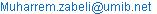(M. Zabeli)

Muharrem Zabeli, Zarife Bajraktari-Gashi, Ahmet Haxhiaj. Determination and Calculation of Components Cargo (Slag) During Smelting of Copper Ores. International Journal of Mineral Processing and Extractive Metallurgy. Vol. 1, No. 3, 2016, pp. 14-18. doi: 10.11648/j.ijmpem.20160103.11

Received: April 14, 2016; Accepted: May 10, 2016; Published: June 7, 2016

Abstract: After smelting the copper ores in flame furnace crated matte that is smelting Cu2S and FeS. The amount of copper in Matte determined amount of Cu2S while rest is while FeS. In reallity matte contains less sulfur and other metals such as: PbS, ZnS, Fe3O4 and noble metals but their amount is small and does not affect the calculation. Processes of smelting in furnace and also in the converter is conditional on the fact that sulfur (S) is greater affinity to copper (Cu) than to iron (Fe). The percentage of copper in Matte depends on the amount of sulfur in cargo. Copper associated with assigned amount of sulfur and forms Cu2S while the rest non-oxidized sulfur forms FeS. It follows that, much more have sulfur much more will be formed with FeS with that reduced the amount of copper in Matte. The amount of sulfur to oxidize the whole amount of sulfur in cargo ranges from 13-30% and passes in the form of SO2 in gases. From the amount of iron in cargo an part passes Matte in form of FeS while the rest in slag in form of FeO. Losses of copper in slag are less than 0.9% of the amount of slag that with more precise definition of cargo and control of technological parameters of the process can be reduced.

Keywords: Cargo, Smelting, Matte, Slag

1. Theoretical Basis of the Process of Smelting and the Main Chemical Reactions

About 80 % of primary copper production comes from low-grade or poor sulfide ores. After enrichment steps, the copper concentrates are usually treated by pyrometallurgical methods.

Generally, copper extraction follows the sequence (see Figure below):

1. Beneficiation by froth flotation of ore to give copper concentrate

(Optional partial roasting to obtain oxidized material or calcines)

2. Two-stage pyrometallurgical extraction

1. smelting concentrates to matte

2. converting matte by oxidation to crude (converter or blister) copper

3. Refining the crude copper, usually in two steps

1. pyrometallurgically to fire-refined copper

2. electrolytially to high-purity electrolytic copper

The melting process develop due to the heat that is released by burning the fuel, so that the combustion process is one of the basic conditions and normal economic functioning furnace. As fuel used coal powder which after burning must ensure:

The maximum benefit amount of heat and its use as the best

smelting temperature sufficient to fully cargo

To achieve the maximum temperature in the furnace, namely to ensure a better functioning of the furnace must be provided:

Fuel qualitative

Appropriate cartridge furnace and its elements

Better control of the combustion process

Careful adjustment of the capacity of the furnace etc.

Smelting chemical reactions consist of diffraction sulfur reactions and in the formation of slag reactions:

2CuFeS2 →Cu2S+2FeS+S

FeS2→FeS+S

2CuS→Cu2S+S

3Fe2O3→2Fe3O4+0.5O2

Cu2O+FeS→ Cu2S+FeO

2Fe3O4+3SiO2=3[(FeO)2xSiO2]+O2

CaO+SiO2→ CaOxSiO2

3Fe3O4+FeS+ 5SiO2=5[(FeO)2xSiO2]+SO2

2FeS+6Fe2O3+7SiO2=7[(FeO)2xSiO2]+2SO2

If Mette considered as a three-component system of copper, iron, and sulfur without considering other filth, then its properties can be studied with the analysis of three-component diagram Cu-Fe-S.

The main properties of smelting slag as a system dependent on the chemical composition. Physical properties of the slag with particular importance are:

Smelting temperature

Viscosity and

Specific density

Slag mainly composed of three main oxides: SiO2, FeO and CaO.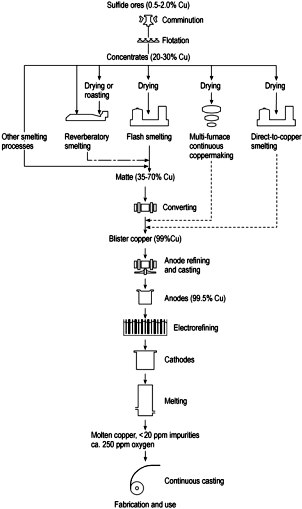Figure 1. Overview of copper production.

Combustion process gases during their passage through the furnace, draw away a significant amount of ash and fine particles cargo, therefore it should be noted that we do not need to own furnace on the gas pressure. The temperature of the furnace gases found in the range of 1300-1350oC and depends on the smelting temperature of the slag. Mainly containing gases: CO2, SO2, CO and O2.

The capacity of the furnace, namely the amount of cargo to be processed per unit of time and the surface of the furnace depends on these factors:

Temperatures with which enters the furnace and cargo

Heat to dissolve the amount of cargo which is obtained by burning fuel (taking into account heat losses)

Determining accurate as cargo elements for smelting

Better control of the working process (mode of operation of the furnace better), which provides minimal loss of copper slag.

2. Determination of Cargo Components

Determination of cargo to gain assigned composition of matte and slag done in this way:

• First determined to taken 100 part of drying ore concnetrating which will be the main ingredient of cargo

• Then assigned the amounts of the other components needed to mix

Concentrating ore usually have no sulfur to obtain the requested matte. It meets next ore of non-concentrating. Non-concentrating ore it contains an amount of copper which is associated with copper ore that conentrating.

After this by the ratio of slag determined amount of iron ore and amount of limestone (CaO) under given conditions.

3. Data from Experimental Work

The composition of ores that are prepared for smelting in flame furnace and limestone, according to laboratory analysis are given in the table:

Table 1. Composition of ores that are prepared for smelting.

 Ore of concentration A Composition in % Ore of Non-concentration B Composition in % Ore of Iron C Composition in % Limestone D Composition in % Cu2S 14% CuFeS2 30% Fe2O3 80% Fe2O3 80% FeS 10% FeS2 20% SiO2 12% SiO2 15% Fe2O3 31% SiO2 50% Al2O3 3% Al2O3 3% SiO2 36% CaCO3 5% CaCO3 2% Al2O3 9%

As combustibles, furnace use coal powder in amounts of 13 parts coal powder to 100 parts cargo. Coal powder containing 15% ashes with composition: 90% SiO2 and 10% FeO.

From practical experience obtained that an amount of ashes (approximately half) remain in cargo while the rest leave with gases.

Also from practical experience obtained by the amount of 20% sulfur of cargo goes with gases (this amount is taken from the process gas analysis)

In this paper determined and calculated cargo of 1000 kg (composed by mixing the three ore, limestone and combustibles) to gain the Matte with 42% copper and slag ratio:

SiO2, CaO, FeO = 40:15:45 since wheras MgO calculated as CaO in equivalent ratio.

It is worth mentioning that the amounts mattes having copper (40-45%) Cu and slag with high iron ratio to enable an optimal work (with suitable parameters such as viscosity, tepmperatura etc.) of furnace for smelting.

4. Analytical Calculations

Knowing that matte (m=Cu2S+FeS) contains 42 % Cu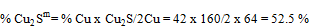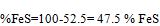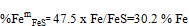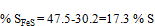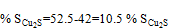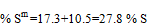These data calculated ratio obtained mattes

Cu:Fe:S = 42:30.2:27.8

For 100 kg of ore cocnentration (A) has:

CuA = 100 x 0.14x2Cu/Cu2S = 11.2 kg Cu

42 kg Cu: 27.8 kg S = 11.2 kg Cu:x

x=7.41kg S

SA=100(0.14 x S/Cu2S+0.10 x S/FeS) x (1-0.2)=5.15 kg S

Explanation: (1-0.2) taken that amount of S 20 % that passes in gases.

Amount of sulfur (S) from ore B should be:

SB=7.41-5.15=2.26 kg S

For 1kg of ore B:

SB1kg = 1x(0.3 x 2S/CuFeS2+0.2x2S/FeS2) x 0.8=0.169 kgS / kgB

Cu/1 kgB=0.3 x Cu/CuFeS2=0.3 x 64/184=0.1043 kgCu / kgB

42 kgCu:27.8 kgS=0.1043:y

y=0.1043 x 27.8/42=0.069 kgS

SB→A=0.169-0,069=0.1 kgS / kgB

1 kgB:0.1 kgS=B:2.26 kgS

B=1 x 226/0.1=22.6 kgB/100 kgA

100 kgA+22.6 kgB

SiO2A+B=36+22.6 x 0.5=47.3 kgSiO2

FeA=10 x Fe/FeS+31 x 2Fe/Fe2O3=28.10 kg Fe

FeB=22.6(0.3 x Fe/Fe2O3+0.2 x Fe/FeS2)=4.4 kgFe

∑Fe=28.10+4.4=32.5 kgFe

CuA+B=11.2+22.6 x 0.1043=13.56 kgCu

Fem=13.56 x 30.2/42=9.8 kgFe

Fez=FeA+B- Fem=32.5-9.8=22.7 kgFe from A+B

FeO=22.7 x FeO/Fe=29.2 kgFeO

From report of slag has:

40 kgSiO2:45 kgFeO=47.3 kgSiO2A+B: x

Explanation: from 100 kg A+22.6 kgB=100 x 0.36+22.6 x 0.5=36+11.3=47.3

x=53.2 kgFeOz

FeOc=53.2-29.2=24 kgFeO

From fraction: Fe2O3→2FeO+1/2O2

Ore C contain 80 % Fe2O3:

1 kgCxFeO / kgC=0.8 x 2FeO/Fe2O3=0.72 kg C

Ore C contain SiO2, that amount of FeO for SiO2 own smelting is:

40 kg SiO2:45 kgFeO=0.12 kg : x

x=45 x 0.12/40=0.135 kgFeO

FeOC→(A+B)= 0.72-0.135 = 0.585 kgFeO / kgC

1 kgC:0.585 kgFeO=C:24 kg

C=41 kg ore C

SiO2A+B+C=47.3+41 x 0.12=52.2 kgSiO2

40 kgSiO2:15 kgCaO=52.2 kgSiO2:x

x=15 x 52.2/40=19.6 kgCaO

CaOC=41 kg x 0,05 x CaO/CaCO3=1.1 kgCaO

CaOD=19.6-1.1=18.5 kg

CaO+MgO/kgD=1 x 0.8 x CaO/CaCO3+0.15 x MgO/MgCO3 x CaO/MgO=0.548 kgCaO

Calculate amount of CaO for SiO2 of own limestone:

40:15:0,02:y

y=0,008 kgCaO/0,02 kgSiO2

Amount of CaOD→­(A+B+C+D)=0.548-0,008=0.54 kgCaO/kgD

1 kgD:0.54 kgCaO=D:18.5 kg

D=18.5x1/0.54=34.3 kg D

D=34.3 kg

Now should calculate and amount of FeO and CaO for SiO2 that comes from coal ash:

Amount of coal ash uses (q) is:

q=13/100(A+B+C+D)=0.13(100+22.6+41+34.3)

q=25.7 kg

Amount of ashes (H) that falls in cargo is:

H=1/2 x 25.7 x 0.15=1.93 kg ashes

Kg ash contain:

SiO2H=1.93 x 0.90=1.74 kg SiO2

40kgSiO2:45 kgFeO=1.74SiO2:a

a=45 x 1.74/40=1.96 kgFeO

FeOH=1.93 x 0.10=0.19 kgFeO

a'=1.96-0.19=1.77kgFeO- amount of FeO in ash shold be added

1 kgC:0.585 kgFeO=C':1.77 kgFeO

C'=1.77/0.585=3.0 kg

40 kgSiO2:15 kgCaO=1.74 kgSiO2:b

b=15 x 1.74/40=0.65 kgCaO

Amount of CaO that should added in limestone D is:

b'=0.65-0.08=0.57 kgCaO

1 kgD:0.54 kgCaO=D':0.57

D'=1.1 kg

Cargo is:

A=100

B=22.6

∑C=41+3=44

∑D=34.3+1.1=35.4

∑=202 kg

Calculation for 1000 kg =1t of cargo:

202 kg:100A=1000:x=100/202 x 100=495 kgA

22.6 x 1000/202=112 kgB

44 x 100/202=218 kgC

35.4 x 1000/202=175 kgD

∑(A+B+C+D)=1000 kg

5. Discus of Results

The results obtained by calculations analysis for the base are obtained 100 kg of ore that concentrating (A). Per 100 kg of ore that concentrating (A) are calculated quantities of components: ore non-concentrating (B), ore iron (C) and limestone (D), based on the chemical reactions that take place in the area reactive and from the balance material of the process.

The composition of full OF CARGO presented in the table for the quantity of 1000 kg = 1t cargo

Table 2. Quantitaive compositon of cargo for 1000 kg=1t.

 A B C D Cargo 495 112 218 175 ∑1000 kg

Table 3. Percentage of cargo components.

 A B C D Cargo 49.5 11.2 21.8 17.5 ∑100 %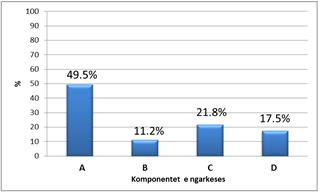Figure.2. Percentage of cargo components.

6. Conclusions

Technological main indicators characterizing the operation of the furnace for smelting are:

Quantity of cargo to melt expressed in t / 24h

Consume of fuel expressed as a percentage of the mass of the cargo molten

Copper ladder crossing in matte (minimum copper losses).

The results achieved and their practical application to fuse the copper ores can be concluded that during the work will have suitable parameters that enable continual good job of merging such as:

The composition of mattes that Cu

Viscosity of mattes that Cu

The composition of slag

Viscosity of slag

Minimal loss of Cu

To achieve optimal values of main parameters working during smelting of the cargo, the main factors is the calculation and determination of the amount of cargo elements, which can value that is achieved in this paper.

References

1. AGOLLI F. (1985) Metalurgjia e metaleve me ngjyrë, UP Prishtinë.
2. R.Vračar, Ž.Kamberović, D.Sinadinović, V.Savović, S.Stopić, K.Cerović, (2000),Proračuni u metalurgiji obojenih metala, Bakar-Bor
3. Ullmann's Encyclopedia of Industrial Chemistry, 2001)
4. G.Diaz, G.Landelt,A.Luaraschi and C.J.Newman, (1991) Pyrometallurgy of Copper; Vol. 4 of the Proceedings of the Copper 91-Cobre 91 International Symposium: Ottawa, Canada
5. William G. Davenport, Matthew J. King, Mark E. Schlesinger, A.K. Biswas (2002),Extractive Metallurgy of Copper, fourth edition.
6. F.Habashi, (1984)Principles of extractive metallurgy, McGraw Hill, New York, vol 3.
7. T.Rosenkqvist, (1994),Principles of extractive metallurgy, McGrawHill, New York.
8. Zander, M., i dr. (2011) Improving Copper Recovery from Production Slags by Advanced Stirring Methods. u: Copper, General Pyrometallurgy, Vessel Integrity, Process Gas Treatment, vol. 1
9. Biswas, A.K. and Davenport, W.G. (1994) Extractive Metallurgy of Copper, 3"' Edition, Elsevier Science Press, New York, NY.
10. Shimpo, R., Goto, S., Ogawa, 0. and Asakura, I. (1986) A study on the equilibrium between copper matte and slag. Can. Metall. Quart., 25, 113 121
11. Mark E. Schlesinger,Matthew J. King, Kathryn C. Sole, William G. Davenport.(2011) Extractive Metallurgy of Copper, Fifth Edition.

 Contents 1. 2. 3. 4. 5. 6.
Article ToolsAbstractPDF(752K)